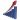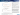# Where Lease Rates are Going in a Higher Interest Rate Environment# Where Lease Rates are Going in a Higher Interest Rate Environment## Where Lease Rates are Going in a Higher Interest Rate Environment• Interest Rates and Lease Rentals per month correlate strongly against one another and are positive in orientation (as interest rates increase, lease rentals also increase)
• Using A320-200 new build aircraft (years 1998 to 2017), adjusted monthly lease rates using CPI; thus adjustment is in 2017 US dollars
• Overlaid lease rates with one-year Libor rates (no lags in the data) to establish LR vs. Interest rate relationship
• Top Chart indicates the relationship; simple straight correlation analysis show a .81 correlation meaning that 81% of the change in lease rate is due to interest rate changes
• The lower chart indicates a simple regression analysis of monthly lease rates using interest rates as the independent variable which shows the fit between the regression projection vs. actual lease rates; a little disconnect is apparent in the last couple of periods analyzed as competitive pressures appears to be overwhelming where rates should be trending
• In this analysis, for each one point change in one-year Libor, monthly lease rate increases by \$20K per month; if interest rates climb to their 2007 levels, monthly lease rates would increase about \$80K from 2017 levels